International
Tables for
Crystallography
Volume B
Reciprocal space
Edited by U. Shmueli

International Tables for Crystallography (2010). Vol. B, ch. 1.5, pp. 180-181   | 1 | 2 |

## Section 1.5.4.3. Wintgen positions

M. I. Aroyoa* and H. Wondratschekb

aDepartamento de Fisíca de la Materia Condensada, Facultad de Cienca y Technología, Universidad del País Vasco, Apartado 644, 48080 Bilbao, Spain , and bInstitut für Kristallographie, Universität, D-76128 Karlsruhe, Germany
Correspondence e-mail:  wmpararm@lg.ehu.es

#### 1.5.4.3. Wintgen positions

| top | pdf |

In order to avoid confusion, in the following the analogues to the Wyckoff positions ofwill be called Wintgen positions of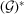; the coordinates of the Wyckoff position are replaced by the k-vector coefficients of the Wintgen position, the Wyckoff letter will be called the Wintgen letter, and the symbols for the site symmetries ofare to be read as the symbols for the little co-groups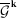of the k vectors in. The multiplicity of a Wyckoff position is retained in the Wintgen symbol in order to facilitate the use of IT A for the description of symmetry in k space. However, it is equal to the multiplicity of the star of k only in the case of primitive lattices.

In analogy to a Wyckoff position, a Wintgen position is a set of orbits of k vectors. Each orbit as well as each star of k can be represented by any one of its k vectors. The zero, one or two free parameters in the k-vector coefficients correspond to special k vectors, i.e., they define symmetry points, symmetry lines or symmetry planes. Three free parameters indicate general k vectors. The different stars of a Wintgen position are obtained by varying the free parameters.

For convenience, we call the set of all k vectors of a Wintgen position a k-vector type. A k-vector type consists of complete orbits of k vectors and thus of full stars of k vectors; the different orbits of a k-vector type are distinguished by the value(s) of the variable parameter(s) of their k vectors. Correspondingly, the irreps belonging to a k vector are specified by these parameters and the irreps belonging to a k-vector type form a type of irreps, Boyle (1986).

It may be advantageous to describe the different stars belonging to a Wintgen position in a uniform way. For this purpose one can define:

Definition. Two k vectors of a Wintgen position are uni-arm if one can be obtained from the other by parameter variation. The description of the stars of a Wintgen position is uni-arm if the k vectors representing these stars are uni-arm.

The uni-arm description is particularly useful to check whether different sets of k vectors belong to the same k-vector type or not. Because of the shape of the representation domain or of the asymmetric unit, a k-vector type may be split into different parts which belong to different arms of different k-vector stars. A uni-arm description may be obtained by the introduction of flagpoles and wings, see Section 1.5.5.1.

For the uni-arm description of a Wintgen position it is easy to check whether the parameter ranges for the general or special constituents of the representation domain or asymmetric unit have been stated correctly. For this purpose one may define the field of k as the parameter space (point, line, plane or space) of a Wintgen position. For the check one determines that part of the field of k which is in the unit cell. The order of the little co-group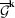(represents those operations which leave the field of k fixed pointwise) is divided by the order of the stabilizer of the field in[which is the set of all symmetry operations mod (integer translations) which leave the field invariant as a whole]. The result gives the independent fraction of the volume of the unit cell or the area of the plane or length of the line.

If the description is not uni-arm, the uni-arm parameter range will be split into the parameter ranges of the different arms. These parameter ranges of the different arms are not necessarily equal, for examples see Section 1.5.5.

Remark. One should avoid the term equivalent for the relation between k vectors of the same type but with different parameters, as used by Stokes et al. (1993) for Λ and F or B, C and J of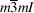, see examples (1)and (2)in Section 1.5.5.4.4. To belong to the same k-vector type is only a necessary, not a sufficient, condition for k-vector equivalence. On p. 95 of BC is the following definition: Two k vectors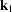and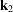are equivalent if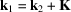, where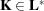'. One can express this by saying: Two k vectors are equivalent if their difference is a vector K of the (reciprocal) lattice'. We prefer to extend this equivalence by saying: `Two k vectorsandare equivalent if and only if they belong to the same orbit of', i.e if there is a matrix partand a vectorbelonging tosuch that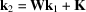, see equation (1.5.3.13). The k vectors of Stokes et al. (1993) are not equivalent under this definition, see Davies & Dirl (1987). If the representatives of the k-vector stars are chosen uni-arm, their non-equivalence is evident.

It must be mentioned that two k vectors of the same type are only called equivalent here if they belong to the same orbit of k vectors, i.e. a k-vector type is not an equivalence class with respect to the definition of equivalence stated in this remark.

There are two main reasons why k vectors of the same type split and then have different labels in CDML:

 (1) The higher the symmetry of the point group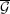of, the higher is the symmetry of the lattice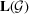and of the reciprocal lattice, and thus of the Brillouin zone of. As the symmetry of the Brillouin zone increases, the choice of the boundaries of the representation domain and any other minimal domain becomes more and more restricted. This is because a symmetry element (rotation or rotoinversion axis, plane of reflection, centre of inversion) cannot occur in the interior of the minimal domain but only on its boundary. For the arithmetic crystal class, for example, all boundary planes, lines and points are fixed, such that all possible minimal domains are equivalent. For lower point-group symmetries of the fundamental regions, the choice of the minimal domain is less restricted but the Brillouin zones may become more complicated and may even belong to different topological types depending on the ratios of the lattice parameters. Faces and lines on the surface of the Brillouin zone may appear or disappear or change their relative sizes depending on the lattice parameters, causing different descriptions of Wintgen positions. This does not happen in unit cells or their asymmetric units. Therefore, as already mentioned in Section 1.5.4.1, BC and CDML preferred to replace the different complicated bodies of the Brillouin zones for the possible values of the lattice parameters of the triclinic and monoclinic lattices by the simple primitive unit cells of the reciprocal lattice and to choose the representation domain correspondingly. (2) For non-holosymmetric space groups the representation domainis a multiple of the basic domain. CDML introduce new letters for k vectors which do not belong to. The more symmetry a space group has lost compared to its holosymmetric space group, the more letters of k vectors are introduced. The symbols of the basic domainare kept in the names referred to the representation domain. In most cases one can make the new k vectors uni-arm to k vectors of the basic domainby an appropriate choice ofand. Then these k vectors belong to the same k-vector type and the additional labels can be avoided by extension of the parameter range in the k-vector space (Boyle, 1986).

Examples where new letters can be avoided by the extension of the parameter range are common, see, e.g., the examples of Section 1.5.5.

In the following example, the introduction of a new name in the transition from a holosymmetric space group to a non-holosymmetric one cannot be avoided because the Wintgen position splits into two positions. We consider the k label Z, α, ½, 0 of CDML for the arithmetic crystal class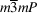, reciprocal-space group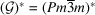, isomorphic to, Wyckoff position 12 h mm2 x, ½, 0. In the subgroup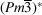, this Wintgen position splits into the two positions α, ½, 0 and ½,α,0, called Z and ZA by CDML. In the description of IT A they are 6 g mm2 x, ½, 0 and 6 f mm2 ½, x, 0. In, they form two different Wyckoff positions and thus need two different names, as do their Wintgen positions.

### References

Boyle, L. L. (1986). The classification of space group representations. In Proceedings of the 14th International Colloquium on Group-Theoretical Methods in Physics, pp. 405–408. Singapore: World Scientific.
Davies, B. L. & Dirl, R. (1987). Various classification schemes for irreducible space group representations. In Proceedings of the 15th International Colloquium on Group-Theoretical Methods in Physics, edited by R. Gilmore, pp. 728–733. Singapore: World Scientific.
Stokes, H. T., Hatch, D. M. & Nelson, H. M. (1993). Landau, Lifshitz, and weak Lifshitz conditions in the Landau theory of phase transitions in solids. Phys. Rev. B, 47, 9080–9083.